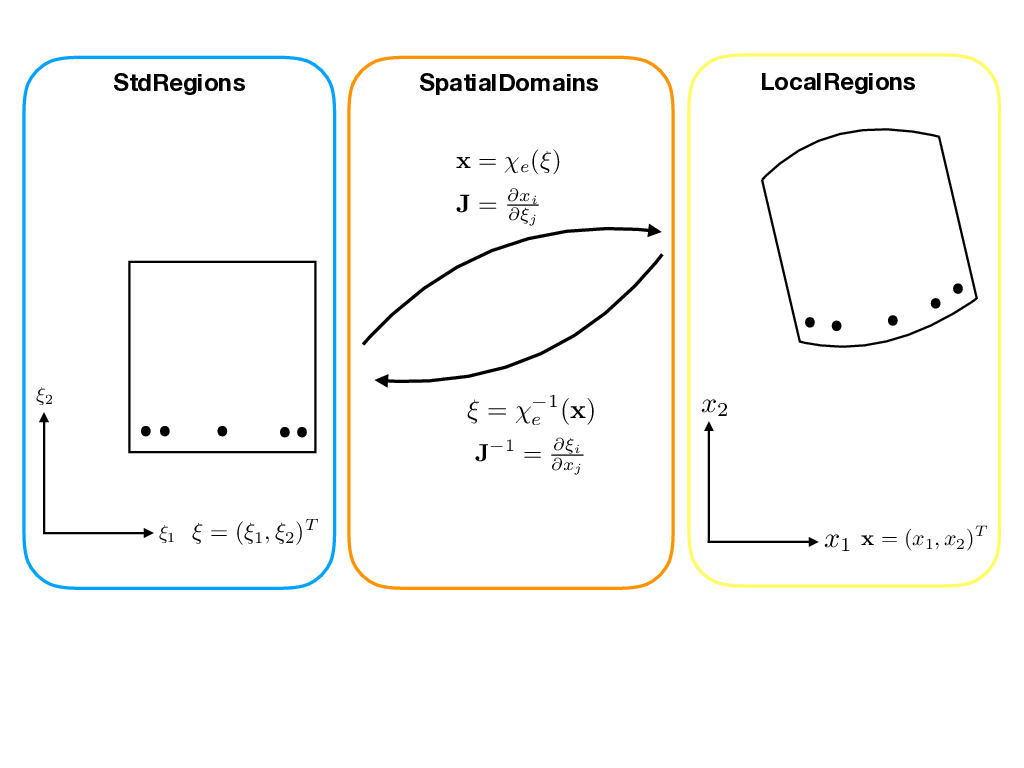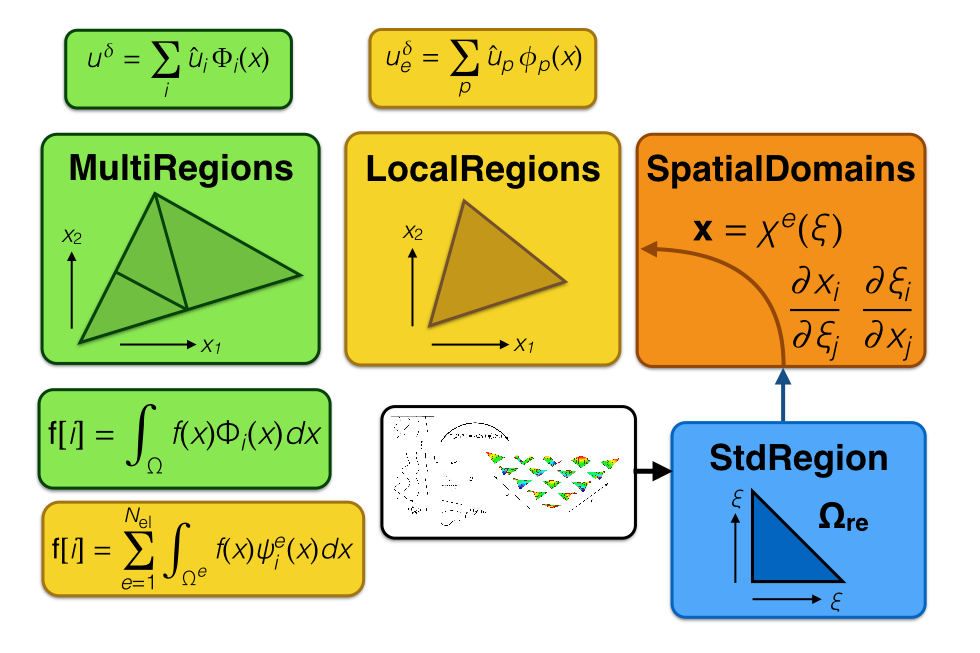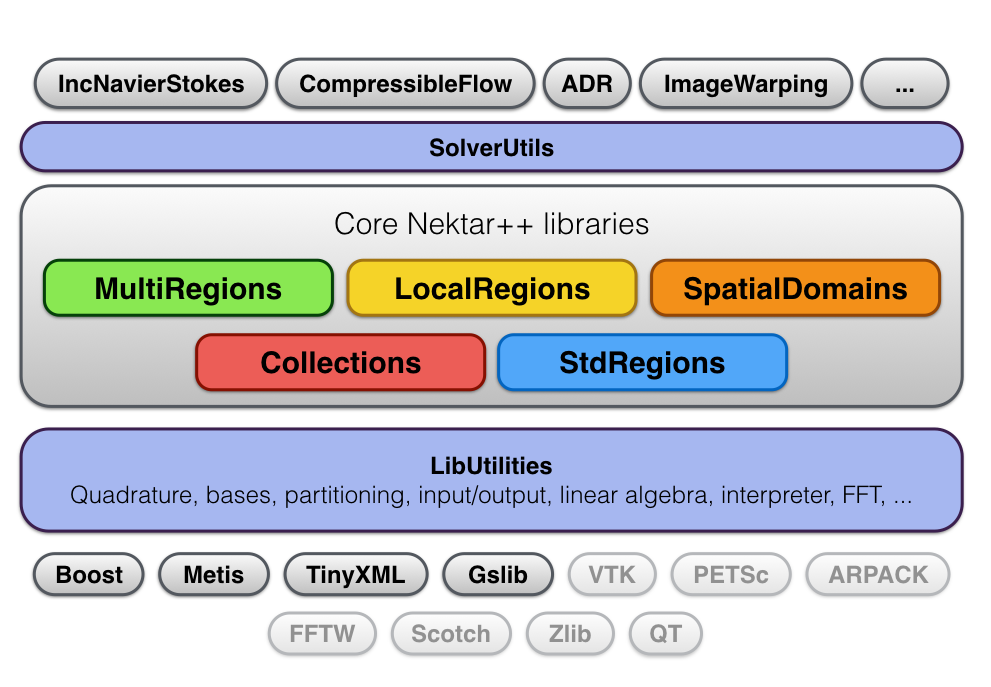### 2.2 The Structure of Nektar++

When we speak of Nektar++, it often means different things to different people, all under an umbrella of code. For the founders of Nektar++, the view was that the core of Nektar++ was the library, and that everything else was to be built around or on top of our basic spectral/hp framework. For others, the heart of Nektar++ are its solvers – the collection of simulation codes, built upon the library, that enable users to solve science and engineering problems. For a smaller group of people, it is all the add-ons that we provide in our utilities, from our mesh generation techniques to our visualization software ideas.

For the purposes of this document, we will structure our discussions into three main parts: library functionality, solvers and utilities. In this section, we will provide an overview of the structure of the libraries. We will start by giving a quick overview of the basic subdirectories contained within library and their purpose. We will then provide a bottom-up description of how the library can be viewed, as well as a top-down perspective. Each perspective (bottom-up or top-down) is fully consistent with each other; the advantage of these approaches is that they help the future developer understand the library as someone trying to build up towards our solvers, or conversely someone trying to understand our solver functionality having already been a solver user and now trying to understand the library components on which it was built.

The basic subdirectories with the library are as follows:

LibUtilities: This library contains all the basic mathematical and computer science building blocks of the Nektar++ code.

StdRegions: This library contains the objects that express “standard region” data and operations. In one dimension, this is the StdSegment. In two dimensions, this is the StdTri (Triangle) and StdQuad (Quadrilateral). In three dimensions, this is the StdTet (Tetrahedra), StdHex (Hexahedra), StdPrism (Prism) and StdPyr (Pyramid). These represent the seven different standardized reference regions over which we support differentiation and integration.

SpatialDomains: This library contains the mesh and elemental geometric information. In particular, this part of the library deals with the basic mesh data structures, and the mapping information (such as Jacobians) from StdRegions to LocalRegions.

LocalRegions: This library contains objects that express data and operations on individual physical elements of the computational mesh. Local regions are spectral/hp elements in world-space (either straight-/planar-sided or curved-sided). Using C++ terminology, a local region object is-a standard region object and has-a spatial domain object.

MultiRegions: This library holds the data structures that represent sets of elements (local regions). At the most fundamental level, these represent the union of local regions into a (geometrically-contiguous) space. By specifying the interaction of these elements, the function space they represent and/or the approximation method is defined. It is at this point in the hierarchy that a set of (local region) elements can be thought of as representing a dG or cG field.

Collections: In this library we amalgamate, in a linear algebra sense, the action of key operators on multiple (standard region or local region) elements into a single, memory-efficient block. These strategies depend on external factors such as BLAS implementation and the geometry of interest.

GlobalMapping: This library supports the analytical mapping of complex physical domains to simpler computational domains.

NekMeshUtils: This library contains processing modules for the generation (potentially from CAD geometries), conversion and manipulation of high-order meshes.

SolverUtils: This library contains data structures and algorithms which form the basis of solvers, or provide auxiliary functionality.

UnitTests: This part of the library contains unit tests that allow us to verify the correctness of the core functionality within Nektar++. These are useful for verifying that new additions or modifications to the lower-levels of the code do not compromise existing functionality or correctness.

##### Bottom-Up Perspective

The bottom-up perspective on the library is best understood from Figures 2.12.2. In Figure 2.1, we take the view of understanding the geometric regions over which we build approximations. Our starting point is within the StdRegions library, in which we define our canonical standard regions. There are seven fundamental regions supported by Nektar++: segments (1D), triangles and quadrilaterals (2D) and tetrahedra, hexahedra, prisms and pyramids (3D). Since we principally employ Gaussian quadrature, these regions are defined by various tensor-product and collapsing of the compact interval [-1,1]. For the purposes of illustration, let us use a quadrilateral as our example. The StdQuadExp is a region defined on [-1,1] × [-1,1] over which we can build approximations ϕe12). Typically ϕe is based upon polynomials in each coordinate direction; using linear functions in both directions yields the traditional Q(1) space in traditional finite elements.Figure 2.1 Diagram showing the LocalRegion (yellow) is-a StdRegion (blue) which has-a SpatialDomain (orange) in terms of coordinate systems and mapping functions. This diagram highlights how points (positions in space) are mapped between different (geometric) regions.

Since ϕe12) lives on Q = [-1,1] × [-1,1] (i.e. ϕe : Q→ ℝ) and is for this example polynomial, we can integrate it exactly (to machine precision) using Gaussian integration, and we can differentiate it by writing it in a Lagrange basis and forming a differential operator matrix to act on values of the function evaluated at points. If the function were not polynomial but instead only a smooth function, we could approximate it with quadrature and decide an appropriate basis by which to approximate its derivatives. All the routines needed for differentiating and integrating polynomials over various standard regions are contained within the StdRegions directory (and will be discussed in Chapter 5). A local region expansion, such as a basis defined on a quadrilateral element, QuadExp, is a linear combination of basis functions over its corresponding standard region as mapped by information contained within its spatial domain mapping. Local region class definitions are in the LocalRegions directory (and will be discussed in Chapter 7). Using the inheritance language of C++, we would say that a local region is-a standard region and has-a spatial domain object. The SpatialDomains directory contains information that expresses the mapping function χe(⋅) from the standard region to a local region. SpatialDomains is explored in Chapter 6. In the case of our quad example, the SpatialDomain object held by a QuadExp would connect the local region to its StdRegion parent, and correspondingly would allow integration and differentiation in world space (i.e., the natural coordinates in which the local expansion lives). If E denotes our geometric region in world space and if F : E → ℝ is built upon polynomials over its standard region, then we obtain F(x1,x2) = ϕee-1(x1,x2)). Note that even though ϕe is polynomial and χe is polynomial, the composition using the inverse of χe is not guaranteed to be polynomial: it is only guaranteed to be a smooth function.

Putting this in the context of MultiRegions, we arrive at Figure 2.2. The MultiRegions directory (which will be discussed in Chapter 9) contains various data structures that combine local regions. One can think of a multi-region as being a set of local region objects in which some collection of geometric and/or function properties are enforced. Conceptually, one can have a set of local region objects that have no relationship to each other in space. This is a set in the mathematical sense, but not really meaningful to us for solving approximation properties. Most often we want to think of sets of local regions as being collections of elements that are geometrically contiguous – that is, given any two elements in the set, we expect that there exists a path that allows us to trace from one element to the next. Assuming a geometrically continuous collection of elements, we can now ask if the functions built over those elements form a piece-wise discontinuous approximation of a function over our Multiregion or a piece-wise continuous C0 approximation of a function over our Multiregion.Figure 2.2 Diagram showing how a MultiRegion (green) contains a collection of LocalRegions (yellow), where a LocalRegion is-a StdRegion (blue) which has-a SpatialDomain (orange) in terms of coordinate systems and mapping functions. This diagram highlights the expansions of the solution formed over each geometric domain.

##### Top-Down Perspective

The top-down perspective on the library is best understood from Figure 2.3. From this perspective, we are interested in understanding Nektar++ from the solvers various people have contributed.Figure 2.3 Diagram showing the top-down perspective on library components. Various solvers (along the top) can capitalize on generic SolverUtils and are built upon the core Nektar++ libraries consisting of MultiRegions (green), Collections (red), LocalRegions (yellow), SpatialDomains (orange) and StdRegions (blue). The most generic mathematical and computer science features are contained within LibUtilities, which in part draws from various community resources such as Boost, Metis, etc.